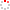#CodeStepByStep## diceSum

Language/Type: C++ backtracking recursion

Write a recursive function named `diceSum` that accepts two parameters: an integer representing a number of 6-sided dice to roll, and a desired sum, and output all possible combinations of values that could appear on the dice that would add up to exactly the given sum. For example, the call of `diceSum(3, 7);` should print all combinations of rolls of 3 dice that add up to 7:

```{1, 1, 5}
{1, 2, 4}
{1, 3, 3}
{1, 4, 2}
{1, 5, 1}
{2, 1, 4}
{2, 2, 3}
{2, 3, 2}
{2, 4, 1}
{3, 1, 3}
{3, 2, 2}
{3, 3, 1}
{4, 1, 2}
{4, 2, 1}
{5, 1, 1}
```

If the number of digits passed is 0 or negative, or if the given number of dice cannot add up to exactly the given sum, print no output. Your function must use recursion, but you can use a single `for` loop if necessary.

Type your C++ solution code here:

This is a function exercise. Write a C++ function as described. Do not write a complete program; just the function(s) above.